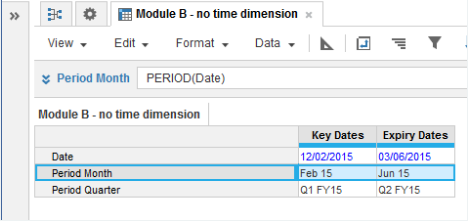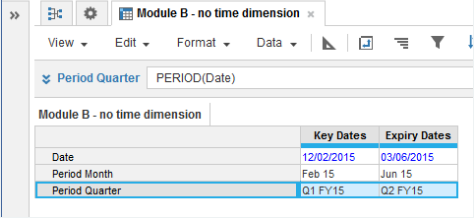1. Calculation functions
2. All Functions
3. Time and Date Functions
4. PERIODConverts a date parameter to a time period as result. The time period is determined by the time scale of the result line item.

## Syntax

`PERIOD(x)`

where:

• x: Date

## Format

Input Format Output Format

x: Date

Time period

## Arguments

The function uses the following arguments:

• x: Date: Hard-coded date, date variable, or line item

## Constraints

The function has the following constraints:

• Source must be date-formatted
• Result must be time period-formatted
• Result must fall within the Time bounds of the model

## Excel equivalent

• No Excel equivalent

## Example

`Period Month = PERIOD(Date) `
• where the result (Period Month) is time period-formatted as Month and the Date source line item is formatted as Date.
•`Period Quarter = PERIOD(Date) `
• where the result (Period Quarter) is time period-formatted as Quarter and the Date source line item is formatted as Date.
•